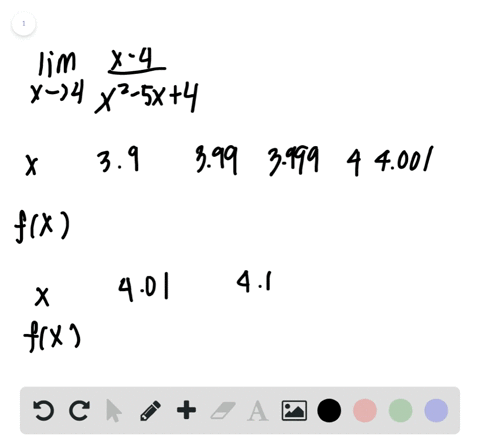Enroll in one of our FREE online STEM bootcamps. Join today and start acing your classes!View Bootcamps## Discussion

You must be signed in to discuss.

## Video Transcript

and this problem were asked, is limit of f of X as X approaches si always equal to FFC fly Or why not? The answer to this claim is no. But we must show that we'll find a counter example to show that this is not sure. So if you look it here at the graph of the function, F of X will compute the limits and in computer off of C and C that the limit as X approaches Earth are seen is actually not going to be equal to FFC. So in this example, let's consider the graph of affects and let's see equal to Earth. No, if we look at the limits as X approaches three from the left and from the right, we'll see at the limit from the left is approaching this wide value here, which is three settlement from the left three as X approaches three no, and then the limit from the right. Coming from this direction is also three. So this tells us that the women exists and the limit is equal to three. However, if you look at the graph and we want to find effort three, you see that that's where the dot is filled in, which is at the Wye Valley. One so half of three is equal to one no, which is not equal to the limit. Thanks approaches. Three. So by this example, we've shown that the limit as X approaches scene for some function F of X is not always necessarily equal to the function value at that point.